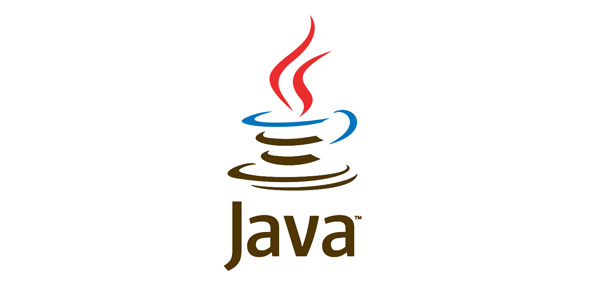# Java Evaluation Quiz Practice Set - 1

10 Questions | Attempts: 310
ShareSettingsThis quiz is conducted as part of the activity based class and its score will be considered for the continuous evaluation of the course "Programming with Java"

• 1.
Which will legally declare, construct, and initialize an array?
• A.

Int [] myList = {"1", "2", "3"};

• B.

Int [] myList = (5, 8, 2);

• C.

Int myList [] [] = {4,9,7,0};

• D.

Int myList [] = {4, 3, 7};

• 2.
Which is a valid keyword in java?
• A.

Interface

• B.

String

• C.

Float

• D.

Unsigned

• 3.
What will be the output of the program?public class Foo{     public static void main(String[] args)    {        try        {            return;        }        finally        {            System.out.println( "Finally" );        }    }}
• A.

Finally

• B.

Compilation fails.

• C.

The code runs with no output.

• D.

An exception is thrown at runtime.

• 4.
What will be the output of the program?public class MyProgram{    public static void main(String args[])    {        try        {            System.out.print("Hello world ");        }        finally        {            System.out.println("Finally executing ");        }    }}
• A.

Nothing. The program will not compile because no exceptions are specified.

• B.

Nothing. The program will not compile because no catch clauses are specified.

• C.

Hello world.

• D.

Hello world Finally executing

• 5.
What is the value of "d" after this line of code has been executed?double d = Math.round ( 2.5 + Math.random() );
• A.

2

• B.

3

• C.

4

• D.

2.5

• 6.
Public class Myfile{    public static void main (String[] args)    {        String biz = args;        String baz = args;        String rip = args;        System.out.println("Arg is " + rip);    }}
• A.

Java Myfile 222

• B.

Java Myfile 1 2 2 3 4

• C.

Java Myfile 1 3 2 2

• D.

Java Myfile 0 1 2 3

• 7.
Which one is a valid declaration of a boolean?
• A.

Boolean b1 = 0;

• B.

Boolean b2 = 'false';

• C.

Boolean b3 = false;

• D.

Boolean b4 = Boolean.false();

• E.

Boolean b5 = no;

• 8.
Which is a valid declarations of a String?
• A.

String s1 = null;

• B.

String s2 = 'null';

• C.

String s3 = (String) 'abc';

• D.

String s4 = (String) '\ufeed';

• 9.
Public interface Foo{    int k = 4; /* Line 3 */}Which three piece of codes are equivalent to line 3?
1. final int k = 4;
2. public int k = 4;
3. static int k = 4;
4. abstract int k = 4;
5. volatile int k = 4;
6. protected int k = 4;
• A.

1, 2 and 3

• B.

2, 3 and 4

• C.

3, 4 and 5

• D.

4, 5 and 6

• 10.
Public class Test { }What is the prototype of the default constructor?
• A.

Test( )

• B.

Test(void)

• C.

Public Test( )

• D.

Public Test(void)

## Related TopicsBack to top
×

Wait!
Here's an interesting quiz for you.